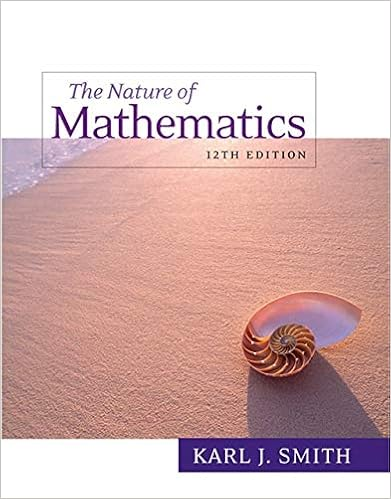# Example 11 factor 81 x 4 y 3 xy 4 solution the terms

• Notes
• 16

This preview shows page 8 - 11 out of 16 pages.

##### We have textbook solutions for you!
The document you are viewing contains questions related to this textbook.The document you are viewing contains questions related to this textbook.
Chapter 5 / Exercise 37
Nature of Mathematics
SmithExpert Verified
Example 11 Factor: 81 x 4 y +3 xy 4 . Solution:
##### We have textbook solutions for you!
The document you are viewing contains questions related to this textbook.The document you are viewing contains questions related to this textbook.
Chapter 5 / Exercise 37
Nature of Mathematics
SmithExpert Verified
The terms are not perfect squares or perfect cubes. However, notice that they do have a common factor. First, factor out the GCF, 3 xy . 81 x 4 y +3 xy 4 =3 xy (27 x 3 + y 3 ) The resulting binomial factor is a sum of cubes with a =3 x and b = y . 81 x 4 y +3 xy 4 ==3 xy (27 x 3 + y 3 )3 xy (3 x + y )(9 x 2 −3 xy + y 2 ) Answer: 3 xy (3 x + y )(9 x 2 −3 xy + y 2 ) When the degree of the special binomial is greater than two, we may need to apply the formulas multiple times to obtain a complete factorization. A polynomial is completely factoredA polynomial that is prime or written as a product of prime polynomials. when it is prime or is written as a product of prime polynomials. Example 12 Factor completely: x 4 −81 y 4 . Solution: First, identify what is being squared. x 4 −81 y 4 =( ) 2 −( ) 2 To do this, recall the power rule for exponents, ( x m ) n = x mn . When exponents are raised to a power, multiply them. With this in mind, we find x 4 −81 y 4 =( x 2 ) 2 −( 9 y 2 ) 2 Therefore, a = x 2 and b =9 y 2 . Substitute into the formula for difference of squares. x 4 −81 y 4 =( x 2 +9 y 2 )( x 2 −9 y 2 ) At this point, notice that the factor ( x 2 −9 y 2 ) is itself a difference of two squares and thus can be further factored using a = x 2 and b =3 y . The factor ( x 2 +9 y 2 ) is prime and cannot be factored using real numbers. x 4 −81 y 4 ==( x 2 +9 y 2 )( x 2 −9 y 2 )( x 2 +9 y 2 )( x +3 y )( x −3 y ) Answer: ( x 2 +9 y 2 )( x +3 y )( x −3 y ) When factoring, always look for resulting factors to factor further. Example 13 Factor completely: 64 x 6 y 6 . Solution: This binomial is both a difference of squares and difference of cubes. 64 x 6 y 6 64 x 6 y 6 ==( 4 x 2 ) 3 −( y 2 ) 3 Difference of cubes ( 8 x 3 ) 2 −( y 3 ) 2 Difference of squares
When confronted with a binomial that is a difference of both squares and cubes, as this is, make it a rule to factor using difference of squares first. Therefore, a =8 x 3 and b = y 3 . Substitute into the difference of squares formula. 64 x 6 y 6 =(8 x 3 + y 3 )(8 x 3 y 3 ) The resulting two binomial factors are sum and difference of cubes. Each can be factored further. Therefore, we have The trinomial factors are prime and the expression is completely factored. Answer: (2 x + y )(4 x 2 −2 xy + y 2 )(2 x y )(4 x 2 +2 xy + y 2 ) As an exercise, factor the previous example as a difference of cubes first and then compare the results. Why do you think we make it a rule to factor using difference of squares first? Try this! Factor: a 6 b 6 −1 Answer: ( ab +1)( a 2 b 2 ab +1)( ab −1)( a 2 b 2 + ab +1) (click to see video) Key Takeaways The GCF of two or more monomials is the product of the GCF of the coefficients and the common variable factors with the smallest power. If the terms of a polynomial have a greatest common factor, then factor out that GCF using the distributive property. Divide each term of the polynomial by the GCF to determine the terms of the remaining factor.
•••